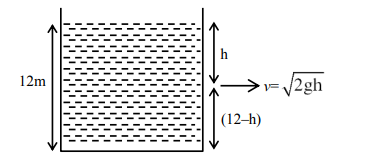# The water is filled upto height of 12 m in a tank having vertical sidewalls.`
Question:

The water is filled upto height of 12 m in a tank having vertical sidewalls. A hole is made in one of the walls at a depth 'h' below the water level. The value of 'h' for which the emerging stream of water strikes the ground at the maximum range is ___ m.

Solution:$\mathrm{R}=\sqrt{2 \mathrm{gh}} \times \sqrt{\frac{(12-\mathrm{h}) \times 2}{\mathrm{~g}}}$

$\sqrt{4 \mathrm{~h}(12-\mathrm{h})}=\mathrm{R}$

For maximum R

$\frac{\mathrm{dR}}{\mathrm{dh}}=0$

$\Rightarrow h=6 m$